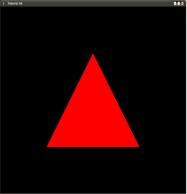modern opengl tutorial

end

# 第五课 Uniform Variables

## Code Walkthru

``glutIdleFunc(RenderSceneCB);``

``````gScaleLocation = glGetUniformLocation(ShaderProgram, "gScale");
assert(gScaleLocation != 0xFFFFFFFF);``````

``````static float Scale = 0.0f;
Scale += 0.001f;
glUniform1f(gScaleLocation, sinf(Scale));``````

``uniform float gScale;``

``gl_Position = vec4(gScale * Position.x, gScale * Position.y, Position.z,1.0);``

## Operation Result